# NCERT Solutions for Class 8 Maths Chapter 15 Introduction to Graphs (EX 15.2) Exercise 15.2

## NCERT Solutions for Class 8 Maths Chapter 15 Introduction to Graphs (EX 15.2) Exercise 15.2

Free PDF download of NCERT Solutions for Class 8 Maths Chapter 15 Exercise 15.2 (EX 15.2) and all chapter exercises at one place prepared by an expert teacher as per NCERT (CBSE) books guidelines. Class 8 Maths Chapter 15 Introduction to Graphs Exercise 15.2 Questions with Solutions to help you to revise complete Syllabus and Score More marks. Register and get all exercise solutions in your emails.

Vedantu also provides NCERT Solutions for all subjects and classes for free. Science Students who are looking for NCERT Solutions for Class 8 Science will also find the Solutions curated by our Master Teachers really Helpful.

Do you need help with your Homework? Are you preparing for Exams?
Study without Internet (Offline)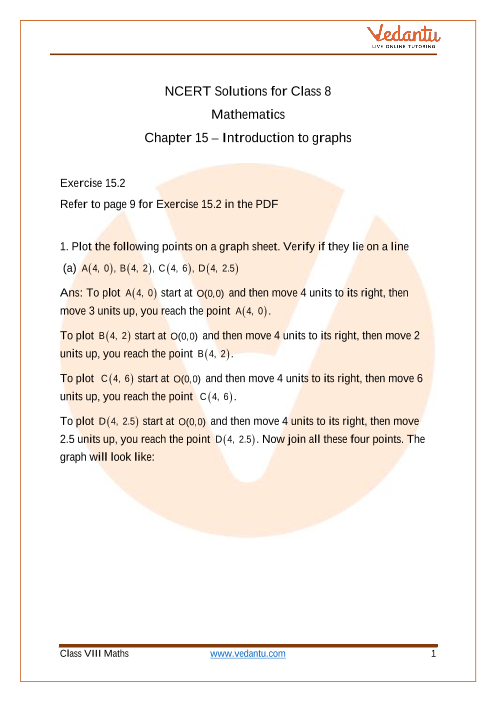Book your Free Demo session
Get a flavour of LIVE classes here at Vedantu## Access NCERT Solutions for Class 8 Chapter 15 – Introduction To Graphs

Exercise 15.2

Refer to page 9 for Exercise 15.2 in the PDF

1. Plot the following points on a graph sheet. Verify if they lie on a line

(a) $A\left( {4,{\text{ }}0} \right),{\text{ }}B\left( {4,{\text{ }}2} \right),{\text{ }}C\left( {4,{\text{ }}6} \right),{\text{ }}D\left( {4,{\text{ }}2.5} \right)$

Ans: To plot $A\left( {4,{\text{ }}0} \right)$ start at $O(0,0)$ and then move 4 units to its right, then move 3 units up, you reach the point $A\left( {4,{\text{ }}0} \right)$.

To plot $B\left( {4,{\text{ }}2} \right)$ start at $O(0,0)$ and then move 4 units to its right, then move 2 units up, you reach the point $B\left( {4,{\text{ }}2} \right)$.

To plot ${\text{ }}C\left( {4,{\text{ }}6} \right)$ start at $O(0,0)$ and then move 4 units to its right, then move 6 units up, you reach the point ${\text{ }}C\left( {4,{\text{ }}6} \right)$.

To plot $D\left( {4,{\text{ }}2.5} \right)$ start at $O(0,0)$ and then move 4 units to its right, then move 2.5 units up, you reach the point $D\left( {4,{\text{ }}2.5} \right)$.

Now join all these four points. The graph will look like:

(Image Will Be Updated Soon)

In the graph , it is obvious that all four points lie on a line.

(b) $P\left( {1,{\text{ }}1} \right),{\text{ }}Q\left( {2,{\text{ }}2} \right),{\text{ }}R\left( {3,{\text{ }}3} \right),{\text{ }}S\left( {4,{\text{ }}4} \right)$

Ans: To plot $P\left( {1,{\text{ }}1} \right)$ start at $O(0,0)$ and then move 1 unit to its right, then move 1 unit up, you reach the point $P\left( {1,{\text{ }}1} \right)$.

To plot $Q\left( {2,{\text{ }}2} \right)$ start at $O(0,0)$ and then move 2 units to its right, then move 2 units up, you reach the point $Q\left( {2,{\text{ }}2} \right)$.

To plot $R\left( {3,{\text{ }}3} \right)$ start at $O(0,0)$ and then move 3 units to its right, then move 3 units up, you reach the point $R\left( {3,{\text{ }}3} \right)$.

To plot $S\left( {4,{\text{ }}4} \right)$ start at $O(0,0)$ and then move 4 units to its right, then move 4 units up, you reach the point $S\left( {4,{\text{ }}4} \right)$.

Now join all these four points. The graph will look like: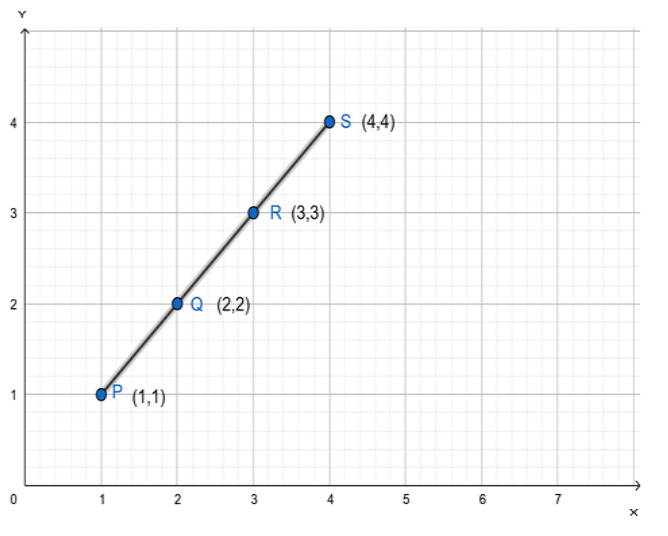In the graph , it is obvious that all four points lie on a line.

(c) $K\left( {2,{\text{ }}3} \right),{\text{ }}L\left( {5,{\text{ }}3} \right),{\text{ }}M\left( {5,{\text{ }}5} \right),{\text{ }}N\left( {2,{\text{ }}5} \right)$

Ans: To plot $K\left( {2,{\text{ }}3} \right)$ start at $O(0,0)$ and then move 2 unit to its right, then move 3 unit up, you reach the point $K\left( {2,{\text{ }}3} \right)$.

To plot $L\left( {5,{\text{ }}3} \right)$ start at $O(0,0)$ and then move 5 units to its right, then move 3 units up, you reach the point $L\left( {5,{\text{ }}3} \right)$

To plot $M\left( {5,{\text{ }}5} \right)$ start at $O(0,0)$ and then move 5 units to its right, then move 5 units up, you reach the point$M\left( {5,{\text{ }}5} \right)$.

To plot $N\left( {2,{\text{ }}5} \right)$ start at $O(0,0)$ and then move 2 units to its right, then move 5 units up, you reach the point$N\left( {2,{\text{ }}5} \right)$.

Now join all these four points. The graph will look like: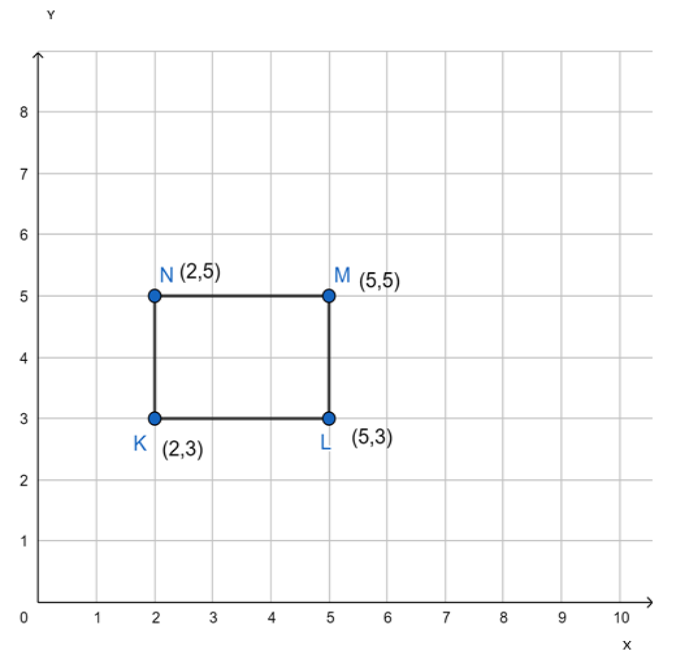Joining all four points gives a rectangle. Thus, all four points do not lie on a line.

2. Draw the line passing through (2, 3) and (3, 2). Find the coordinates of the points at which this line meets the x-axis and y-axis.

Ans: To plot $\left( {2,{\text{ }}3} \right)$start at $O(0,0)$and then move 2 units to its right, then move 3 units up, you reach the point$\left( {2,{\text{ }}3} \right)$.

To plot $(3,2)$ start at $O(0,0)$and then move 3 units to its right, then move 2 units up, you reach the point $(3,2)$.

Now join both the points, and you will get a line segment. Now extend this line segment on both sides to get the line.

The graph will look like: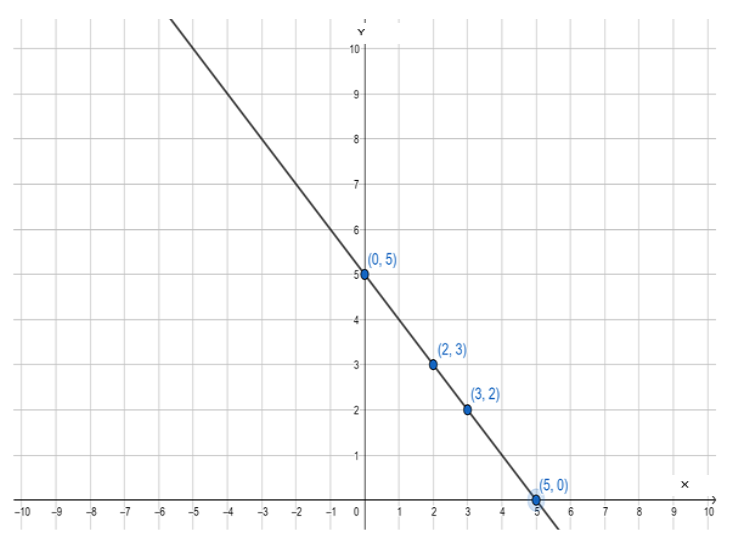In the given graph, one can observe that Coordinates of the points where the  line meets x-axis is $(5,0)$ and y-axis is $(0,5)$.

3. Write the coordinates of the vertices of each of these adjoining figures: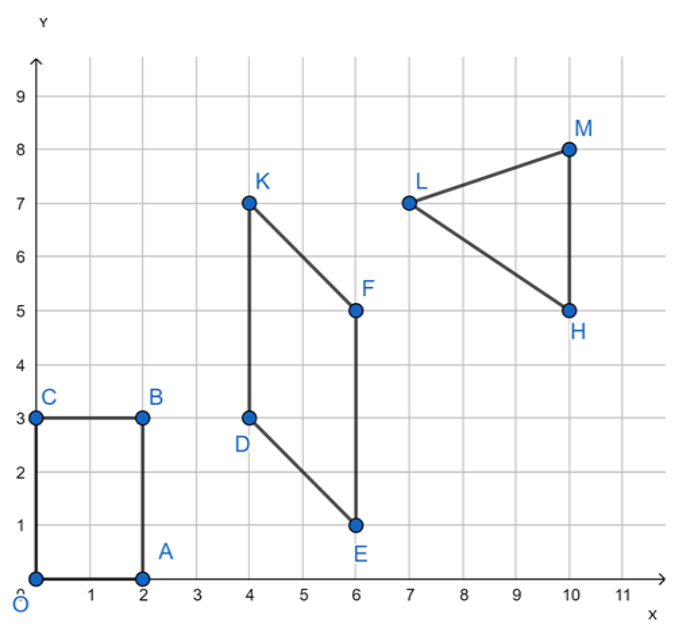Let’s  consider the vertex O:

O is 0 units right to the origin and 0 units up from the origin. Thus, $O(0,0)$.

Let’s first consider the vertex A:

A is 2 units right to the origin and 0 units up from the origin. Thus, $A(2,0)$.

Let’s first consider the vertex B:

B is 2 units right to the origin and 3 units up from the origin. Thus, $B(2,3)$.

Let’s first consider the vertex C:

C is 0 units right to the origin and 3 units up from the origin. Thus, $C(0,3)$.

Let’s first consider the vertex P:

P is 4 units right to the origin and 3 units up from the origin. Thus, $P(4,3)$.

Let’s first consider the vertex Q:

Q is 6 units right to the origin and 1 unit up from the origin. Thus, $Q(6,1)$.

Let’s first consider the vertex R:

R is 6 units right to the origin and 5 units up from the origin. Thus, $R(6,5)$.

Let’s first consider the vertex S:

S is 4 units right to the origin and 7 units up from the origin. Thus, $S(4,7)$.

Let’s first consider the vertex K:

K is 10 units right to the origin and 5 units up from the origin. Thus, $K(10,5)$.

Let’s first consider the vertex L:

L is 7 units right to the origin and 7 units up from the origin. Thus, $L(7,7)$.

Let’s first consider the vertex M:

M is 10 units right to the origin and 8 units up from the origin. Thus, $M(10,8)$.

Thus,

$O{\text{ }}\left( {0,{\text{ }}0} \right),{\text{ }}A{\text{ }}\left( {2,{\text{ }}0} \right),{\text{ }}B{\text{ }}\left( {2,{\text{ }}3} \right),{\text{ }}C{\text{ }}\left( {0,{\text{ }}3} \right){\text{ }}P{\text{ }}\left( {4,{\text{ }}3} \right),{\text{ }}Q{\text{ }}\left( {6,{\text{ }}1} \right),{\text{ }}R{\text{ }}\left( {6,{\text{ }}5} \right),{\text{ }}S{\text{ }}\left( {4,{\text{ }}7} \right){\text{ }}K{\text{ }}\left( {10,{\text{ }}5} \right),{\text{ }}L{\text{ }}\left( {7,{\text{ }}7} \right),{\text{ }}M{\text{ }}\left( {10,{\text{ }}8} \right)$

4. State whether True or False. Correct those are false.

(i) A point whose x coordinate is zero and y-coordinate is non-zero will lie on the y-axis.

Ans: x coordinate is zero implies that the point lies 0 units right to the $O(0,0)$ and y-axis is 0 units right to the $O(0,0)$, i.e., a point with x coordinate 0 will always lie on the y-axis. Therefore, A point whose x coordinate is 0 and y coordinate is non-zero will lie on the y-axis.

Thus, the given statement is true.

(ii) A point whose y coordinate is zero and x-coordinate is 5 will lie on the y-axis.

Ans: y coordinate is zero implies that the point lies 0 units up from the $O(0,0)$ and x-axis is 0 units up from the $O(0,0)$, i.e., a point with y coordinate 0 will always lie on x-axis. Therefore, A point whose y coordinate is 0 and x coordinate is 5 will lie on the x-axis.

Thus, the given statement is false.

(iii) The coordinates of the origin are (0, 0).

Ans: The given statement is true.

## NCERT Solutions For Class 8 Maths Chapter 15 Introduction To Graphs (Ex 15.2) Exercise 15.2

Opting for the NCERT solutions for Ex 15.2 Class 8 Maths is considered as the best option for the CBSE students when it comes to exam preparation. This chapter consists of many exercises. Out of which we have provided the Exercise 15.2 Class 8 Maths NCERT solutions on this page in PDF format. You can download this solution as per your convenience or you can study it directly from our website/ app online.

Vedantu in-house subject matter experts have solved the problems/ questions from the exercise with the utmost care and by following all the guidelines by CBSE. Class 8 students who are thorough with all the concepts from the Subject Maths textbook and quite well-versed with all the problems from the exercises given in it, then any student can easily score the highest possible marks in the final exam. With the help of this Class 8 Maths Chapter 15 Exercise 15.2 solutions, students can easily understand the pattern of questions that can be asked in the exam from this chapter and also learn the marks weightage of the chapter. So that they can prepare themselves accordingly for the final exam.

Besides these NCERT solutions for Class 8 Maths Chapter 15 Exercise 15.2, there are plenty of exercises in this chapter which contain innumerable questions as well. All these questions are solved/answered by our in-house subject experts as mentioned earlier. Hence all of these are bound to be of superior quality and anyone can refer to these during the time of exam preparation. In order to score the best possible marks in the class, it is really important to understand all the concepts of the textbooks and solve the problems from the exercises given next to it.

Do not delay any more. Download the NCERT solutions for Class 8 Maths Chapter 15 Exercise 15.2 from Vedantu website now for better exam preparation. If you have the Vedantu app in your phone, you can download the same through the app as well. The best part of these solutions is these can be accessed both online and offline as well.SHARETWEETSHARESUBSCRIBE